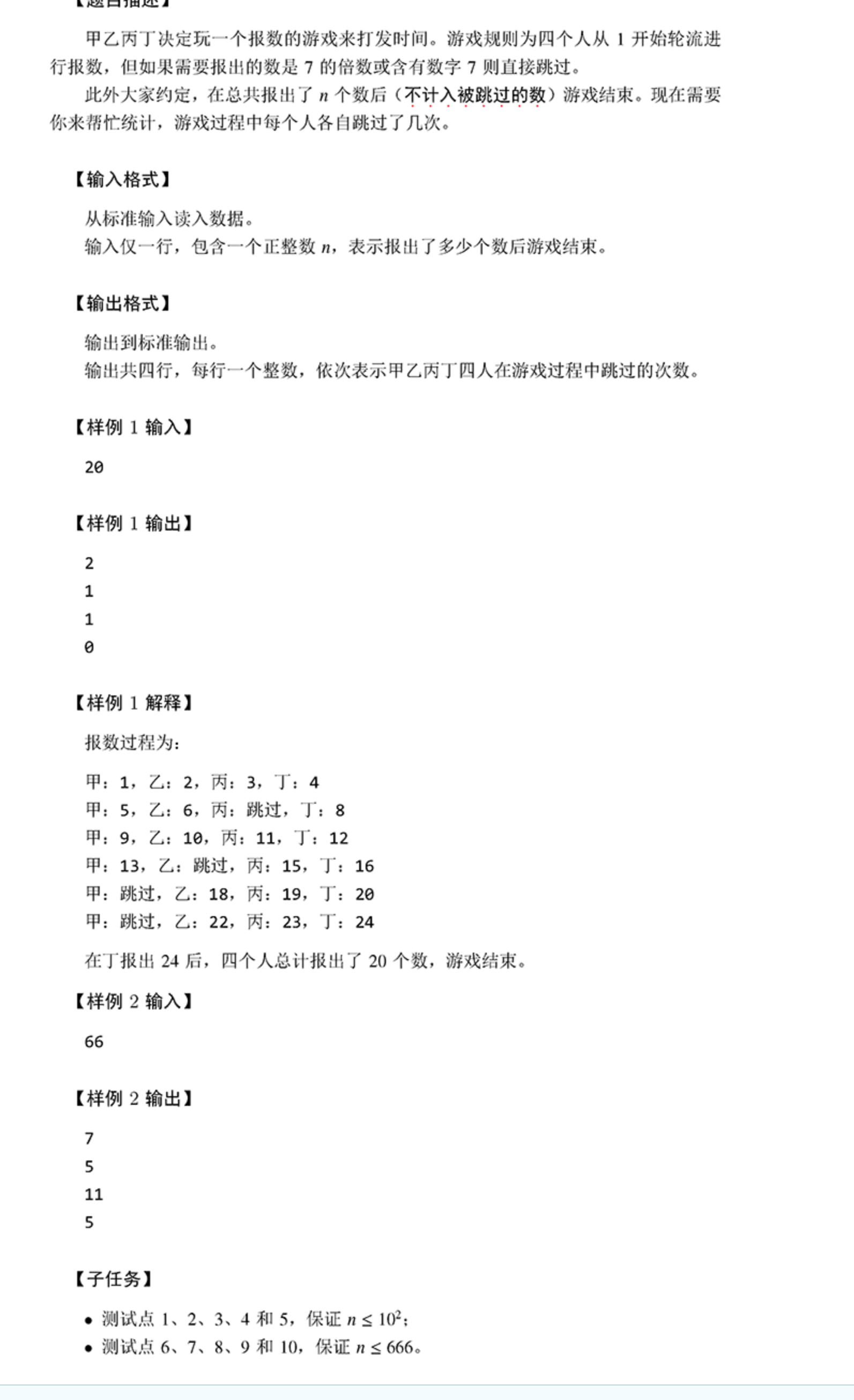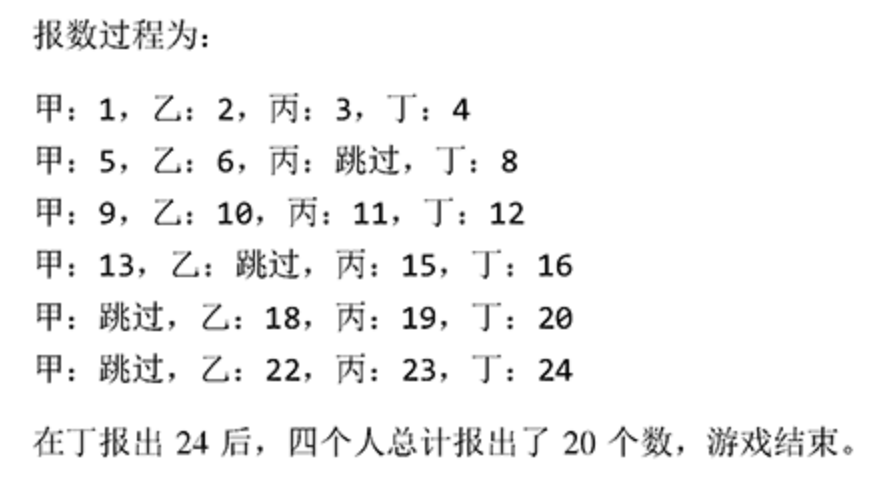# 201912-1报数

## 题目## 思路

 1  arr[k%4]++;7的倍数很简单了，对7取余就行了。

## 代码

  1 2 3 4 5 6 7 8 9 10 11 12 13 14 15 16 17 18 19 20 21 22 23 24 25 26 27 28 29 30 31 32 33 34  /**< 报数 */ #include using namespace std; bool have_seven(int x) { if (x % 7 == 0)return true; else { while (x) { if (x % 10 == 7)return true; x /= 10; } return false; } } int n; int arr; int main() { cin >> n; int m = 1, k = 1; while (m <= n) { if (have_seven(k)) { arr[k % 4]++; } else m++; k++; } for (int i = 1; i <= 3; i++) { cout << arr[i] << endl; } cout << arr; return 0; }